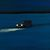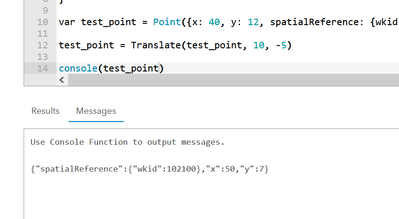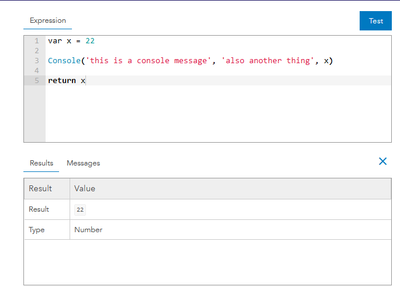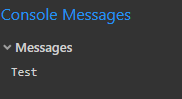# Are Arcade geometry subtypes immutable? (i.e. point, polyline, polygon)

683
7
03-02-2022 10:42 PMby
Frequent Contributor II

If I understand correctly, the Arcade Geometry type has subtypes:

• Point
• Multipoint
• Polyline
• Polygon
• Extent

Question:
Are those subtypes immutable, since the Geometry supertype is immutable?

Geometry is immutable, meaning it is not possible to change the geometry after it is created.

For example, if I assign a value to a point, does that mean that geometry can't be changed?

`var pt = Point({ 'x': 100, 'y': 100, 'spatialReference':{'wkid':102100} });`

1 Solution

Accepted SolutionsbyMVP Regular Contributor

In my experience, yes.

For example, you can't do something like this:

``````var pt = Point({ 'x': 100, 'y': 100, 'spatialReference':{'wkid':102100} });
Console(pt)
// attempt to change x coordinate
// this will fail
pt.x += 5
Console(pt)``````

"Immutable" just means "This object and its values can not be changed".

To work around it, you have to make a copy of the geometry's values, change them and then create a new geometry with these changed values:

``````var pt = Point({ 'x': 100, 'y': 100, 'spatialReference':{'wkid':102100} });
Console(pt)
// attempt to change x coordinate
//pt.x += 5
var new_pt = Point({'x': pt.x + 5, 'y': pt.y, 'spatialReference': pt.spatialReference})
Console(new_pt)

// you could also extract the geometry's dictionary and change the values there:
var pt_dict = Dictionary(Text(pt))
pt_dict.y += 5
var new_pt_2 = Point(pt_dict)
Console(new_pt_2)``````

To change the geometry of polylines or polygons, you have to take it a step further: you have to recreate the paths/rings.

``````var old_path = Geometry(\$feature).paths
var sr = Geometry(\$feature).spatialReference

var new_path = []
for(var i in old_path) {
// move vertext to north east and remove digits, because why not?
var new_vert = Point({'x': Round(old_path[i].x) + 100, 'y': Round(old_path[i].y) + 100, 'spatialReference': sr})
Push(new_path, new_vert)
}
var new_geo = Polyline({'paths': [new_path], 'spatialReference': sr})

Console(Geometry(\$feature))
Console(new_geo)``````

Have a great day!
Johannes
7 RepliesbyMVP Regular Contributor

In my experience, yes.

For example, you can't do something like this:

``````var pt = Point({ 'x': 100, 'y': 100, 'spatialReference':{'wkid':102100} });
Console(pt)
// attempt to change x coordinate
// this will fail
pt.x += 5
Console(pt)``````

"Immutable" just means "This object and its values can not be changed".

To work around it, you have to make a copy of the geometry's values, change them and then create a new geometry with these changed values:

``````var pt = Point({ 'x': 100, 'y': 100, 'spatialReference':{'wkid':102100} });
Console(pt)
// attempt to change x coordinate
//pt.x += 5
var new_pt = Point({'x': pt.x + 5, 'y': pt.y, 'spatialReference': pt.spatialReference})
Console(new_pt)

// you could also extract the geometry's dictionary and change the values there:
var pt_dict = Dictionary(Text(pt))
pt_dict.y += 5
var new_pt_2 = Point(pt_dict)
Console(new_pt_2)``````

To change the geometry of polylines or polygons, you have to take it a step further: you have to recreate the paths/rings.

``````var old_path = Geometry(\$feature).paths
var sr = Geometry(\$feature).spatialReference

var new_path = []
for(var i in old_path) {
// move vertext to north east and remove digits, because why not?
var new_vert = Point({'x': Round(old_path[i].x) + 100, 'y': Round(old_path[i].y) + 100, 'spatialReference': sr})
Push(new_path, new_vert)
}
var new_geo = Polyline({'paths': [new_path], 'spatialReference': sr})

Console(Geometry(\$feature))
Console(new_geo)``````

Have a great day!
JohannesbyMVP Esteemed Contributor

For simple adjustments, you can also convert the original geometry to a dictionary and manipulate it.

``````var pt = Point({
x: 40,
y: 12,
spatialReference: {
wkid: 102100
}
})

var pt_json = Dictionary(Text(pt))

pt_json['x'] = 20

// returns 20
return Point(pt_json)['x']``````

If you wanted, you could even develop your own custom functions around this idea:

``````function Translate(in_point, x_shift, y_shift){
var pt_json = Dictionary(Text(in_point))

pt_json['x'] += x_shift
pt_json['y'] += y_shift

return Point(pt_json)
}

var test_point = Point({x: 40, y: 12, spatialReference: {wkid: 102100}})

return Translate(test_point, -10, 5)``````But that doesn't change the answer that no, you can't manipulate geometry objects in place, only while converting to another object, or overwriting the entire variable with a new object.

- Josh Carlson
Kendall County GISby
Frequent Contributor II

Side question:

In your post, it looks like you are sending an output to the console().

Out of curiosity, what are you doing there? Where are you displaying that value?

Thanks.byMVP Esteemed Contributor

The console is more for debugging or checking on things. Useful for longer, more complex expressions, but you can only see those messages in the Expression Builder interface, such as in AGOL.

When you test an expression, you can see there are the results and the messages.- Josh Carlson
Kendall County GISbyMVP Regular Contributor

but you can only see those messages in the Expression Builder interface, such as in AGOL

I recently learned that you can also see them in Pro:

Verify the expression, then you can click on the button.Have a great day!
JohannesbyMVP Esteemed Contributor

🤯

- Josh Carlson
Kendall County GISby
Frequent Contributor II

I found this post just now, which confirms what @JohannesLindner mentioned.
Where do the Arcade Console messages go to?